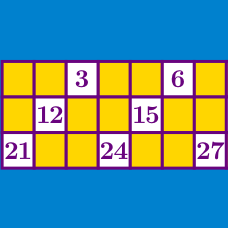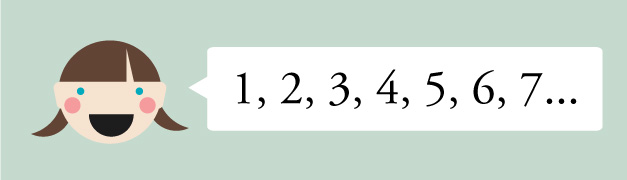Number Theory

# Divisibility: Level 3 Challenges

If the number $\overline{7448x24y}$ is divisible by $72$, and $x \ne y,$ find $x-y$.

${\huge \color{#3D99F6}N = \color{#20A900} {123,4}\color{#D61F06}{F}\color{#20A900}{6,789}}$

If $\color{#D61F06} {F}$ is replaced with a digit from the set (0, 1, 2, 3, 4, 5, 6, 7, 8, or 9) at random, what is the probability that $\color{#3D99F6} {N}$ will be divisible by 3?A longevity chain is a sequence of consecutive positive integers, whose digit sums are never a multiple of 9. What is the longest possible length of a longevity chain?

For similar problems, you can read my note on Construction.

As $n$ ranges over all real values in the interval $[0, 100]$, how many values of $n$ are there such that $4n+ 1$ is an odd integer?

$\begin{array} { l l l l } & && & A & A & A \\ \times & & & & A & A & A \\ \hline &A & A & 8 & 0 & 0 & 1\\ \end{array}$

What is the three digit number $\overline{AAA}?$

×